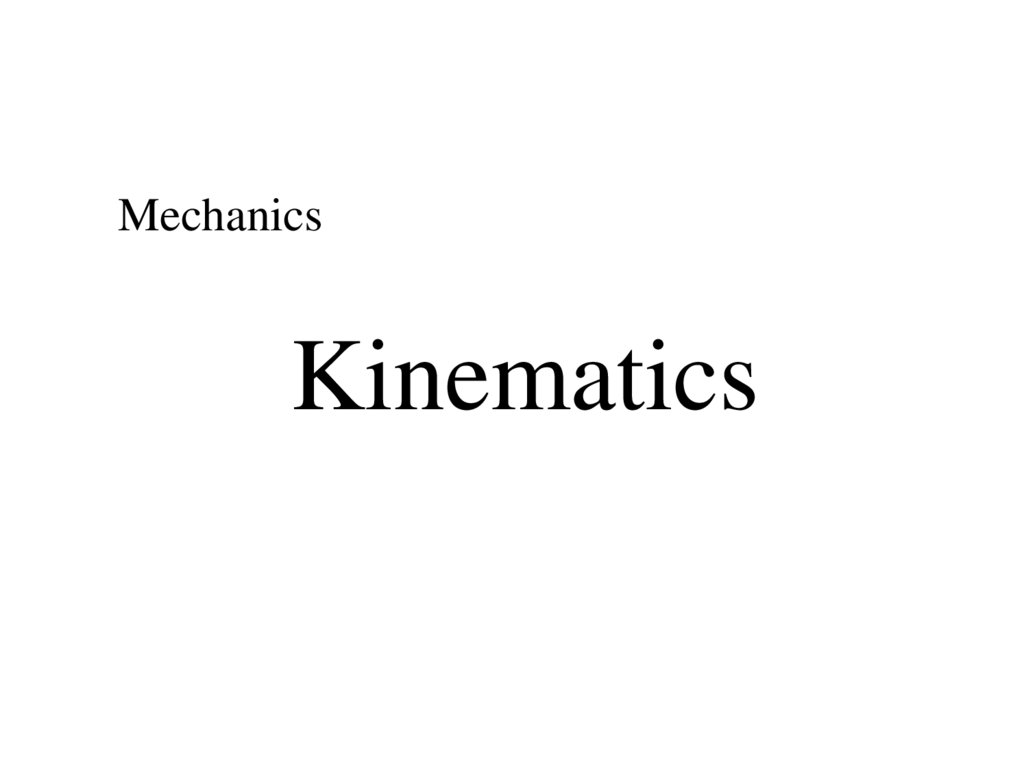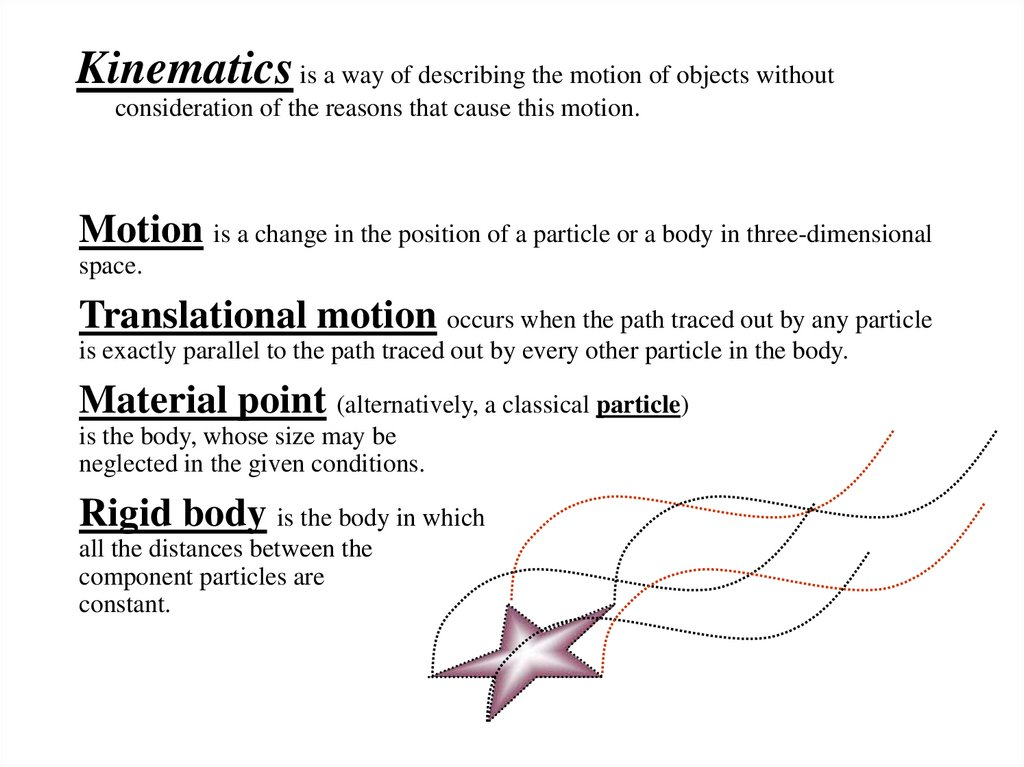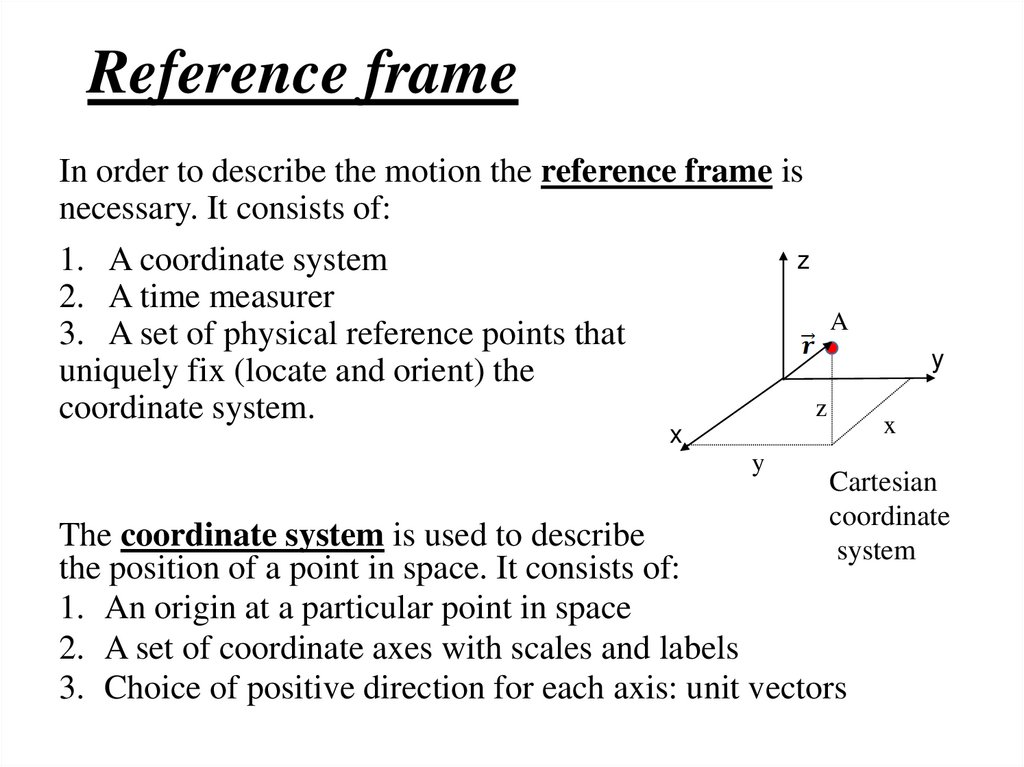# Mechanics. Kinematics

Kinematics

## 2. Kinematics is a way of describing the motion of objects without consideration of the reasons that cause this motion.

Motion is a change in the position of a particle or a body in three-dimensional
space.
Translational motion occurs when the path traced out by any particle
is exactly parallel to the path traced out by every other particle in the body.
Material point (alternatively, a classical particle)
is the body, whose size may be
neglected in the given conditions.
Rigid body is the body in which
all the distances between the
component particles are
constant.

## 3. Reference frame

In order to describe the motion the reference frame is
necessary. It consists of:
z
1. A coordinate system
2. A time measurer
A
3. A set of physical reference points that
uniquely fix (locate and orient) the
z
coordinate system.
x
y
y
x
Cartesian
coordinate
system
The coordinate system is used to describe
the position of a point in space. It consists of:
1. An origin at a particular point in space
2. A set of coordinate axes with scales and labels
3. Choice of positive direction for each axis: unit vectors

## 4.

3 basic kinematic variables:
1. the position of an object is simply its location in
space
2. the velocity of an object is how fast it is changing
its position
3. the acceleration of an object is how fast the
velocity is changing

## 5.

Position and Displacement
Position vector (or radius vector) r is a vector that
points from origin to the object and shows the location of the
object in space.
Position is a function of time.
• units: m, cm, km
Displacement
r is a vector connecting the initial and
the final position of the particle.
Displacement:
r = r 2 – r 1
r1
r2

## 6.

Projections of the vector
y
rx x2 x1
y2
ry
r
y1
0
ry y2 y1
| r | r r
2
x
rx
x1
x2
x
2
y

## 7.

Distance
Trajectory is the line that a moving particle
follows
through space.
Path (distance) l
is the length of the trajectory
line.
• distance is a scalar - “How far”
• units: same as displacement
А
l
r
В

## 8. Velocity

Velocity is the rate of change of the particle’s position vector
with respect to time.
Velocity = displacement
time taken
• velocity is a vector quantity: “How fast and in which
direction”
• direction of the velocity vector is tangent to the trajectory of
the particle
• units: m/s, km/hr
Average velocity
r
v
t

## 9.

Use the laws of vector algebra:
vres v1 v2
| vres | v12 v22
Example:
the resultant velocity
of the swimmer is
determined by the
vector sum of the
swimmer’s velocity
and the river current’s
velocity.

## 10. Speed

Speed is the distance traveled by the particle in unit time.
• speed is a scalar quantity
Speed = distance
• Speed is the magnitude of the velocity
time taken
• it is the rate of change of distance with time
• units: same as velocity
Average speed
l l1 l2 l3
v
t t1 t2 t3
х
l1, t1
l2, t2
l total, t total
l3, t3

## 11. Rectilinear uniform motion

Rectilinear uniform motion: the object travels in a
straight line and covers equal distances in equal intervals of
time.
• Velocity is constant: v const
• Speed is
l
v
t
, where the distance l is covered in time t
Equation for coordinate of the object traveling along the OX
axis:
x x0 vt
S1 1
l x x0 vt
S2 2 5 1 5S1

## 12. Accelerated motion: non-uniform motion when velocity of the object varies with time.

Rectilinear uniformly accelerated motion: the object
travels in a straight line and its velocity increases or decreases by
equal amounts in equal intervals of time.
Acceleration
Acceleration = change in velocity
time taken
• rate of change of velocity with respect to time
– “How fast the velocity is changing”
• acceleration is a vector quantity
• units: m/s/s or m/s2 , m·s-2
v
a
t

## 13.

Equation for velocity of the object during uniformly
accelerated motion:
v v0 at
v0 is the initial velocity of the object, a = const
Equation for coordinate of the object during uniformly
accelerated motion along the OX axis:
2
at
x x0 v0t
2
v 2 v02 2a( x x0 )

## 14. Projectile Motion

Projectile motion is a form of motion in which a body or
particle (called a projectile) is thrown near the Earth's surface,
and it moves under the action of gravity only.
g is the acceleration due to gravity
a g;
g = 9.8 m/s2
If an object is in FREE FALL, gravity will change an objects
velocity by 9.8 m/s every second.
The acceleration due to gravity:
• ALWAYS ACTS DOWNWARD
• IS ALWAYS CONSTANT near the surface of Earth (air
resistance is negligible)

## 15. Motion of the projectile thrown vertically

у
0
0
у
g
0 gt
gt
y y0 0 t
2
g
Maximum height:
2
0 gt
gt 2
y y0 0 t
2
hmax
v02
2g
Time of reaching the
highest point:
v0
t
g
Time of flight:
2 y0
t
g

## 16. Motion of the projectile thrown horizontally

у
ymax
0 x 0
0 y 0
0
g
x 0 const
y gt
v v g t
2
o
0
х
The projectile motion is superposition of
two motions: (1) uniform motion of a
particle under constant velocity in the
horizontal direction and (2) uniformly
accelerated motion of a particle under
constant acceleration (free fall) in the
vertical direction.
2 2
The angle the
velocity vector
makes with the
horizontal:
vy
gt
tg
vx v0

## 17. Motion of the projectile thrown horizontally

Equations for coordinates:
у
ymax
x 0t
0
g
y ymax
0
Horizontal range:
gt 2
2
Equation of the trajectory:
х
l v0t
2
gx
y y0 2
2v0
Time of flight:
2 y0
t
g

## 18. Motion of the projectile thrown at an angle above the horizontal

0 x 0 cos 0
0 y 0 sin 0
у
0 y
g
0
y
0
0
0 x
x
vx v0 cos
vy v0 sin gt
х
v0 sin gt
v v cos (v0 sin gt ) tg
vx
v0 cos
2
o
2
2
vy

## 19. Motion of the projectile thrown at an angle above the horizontal

Equations for coordinates:
у
0 y
0
y
0
0
x v0 cos t
gt 2
y v0 sin t
2
x
g
0 x
х
v sin 2
l
g
v02 sin 2
Maximum height: hmax
2g
Horizontal range:
2
0
Equation of the
trajectory:
gx 2
y tg x 2
2v0 cos 2

## 21. Rotational motion

Rotational motion occurs if every particle in the body moves
in a circle about a single line. This line is called the axis of
rotation.
0
Uniform circular motion describes
the motion of a body traveling a
circular path at constant speed:
1
R
4
2
1 2 3 ...
const
1 2 3
const

## 22. Angular displacement

Angular velocity, also known as angular
frequency
t
The relation between the linear and angular speed:
v R
Period of rotation is the time interval required
for the body to travel one complete circle.
2 R 2 t
T
v
n
Frequency of the circular motion:
1
v
f
T 2 R 2

## 23.

Uniform circular motion is the case
when the body moves at a constant
speed in a circular path, but still
has an acceleration. The velocity
vector of the body is not constant:
it has constant magnitude, but
changing direction.
• The acceleration due to change in
the direction is called
centripetal. It is directed at all
times towards the axis of rotation,
and its magnitude is:
v2
2
ac R v
R

## 24. Example: quantitative determination of v and a from s or How to calculate v and a from s

Frame Time Pos. (m) Vel. (m/s) Acc. (m/s/s)
1
0.00
0.00
2
0.10
0.59
5.90
-23.00
3
0.20
0.95
3.60
-31.00
4
0.30
1.00
0.50
-10.00
5
0.40
0.95
-0.50
-31.00
6
0.50
0.59
-3.60
s
v
v
, a
t
t
t = 0.10 s

## 25. Example:

• Known values:
vinitial = 10 m/s a = 3 m/s2
• Find vfinal
t=3s
v f vi a t 10 m s 3 m s 2 3s 19m/s

## 26. Example: You are driving through town at 12 m/s when suddenly a ball rolls out in front of your car. You apply the brakes and

begin
decelerating at 3.5 m/s/s. How far do you travel before
coming to a complete stop?
v 2 vo2 2a ( x xo )
0 12 2 2( 3.5)( x 0)
144 7 x
x
20.57 m

## 27.

Example:
A stone is dropped at rest from the top of a cliff. It is observed
to hit the ground 5.78 s later. How high is the cliff?
y yo voyt 1 gt 2
2
y (0)(5.78) 4.9(5.78) 2
y -163.7 m
H =163.7m